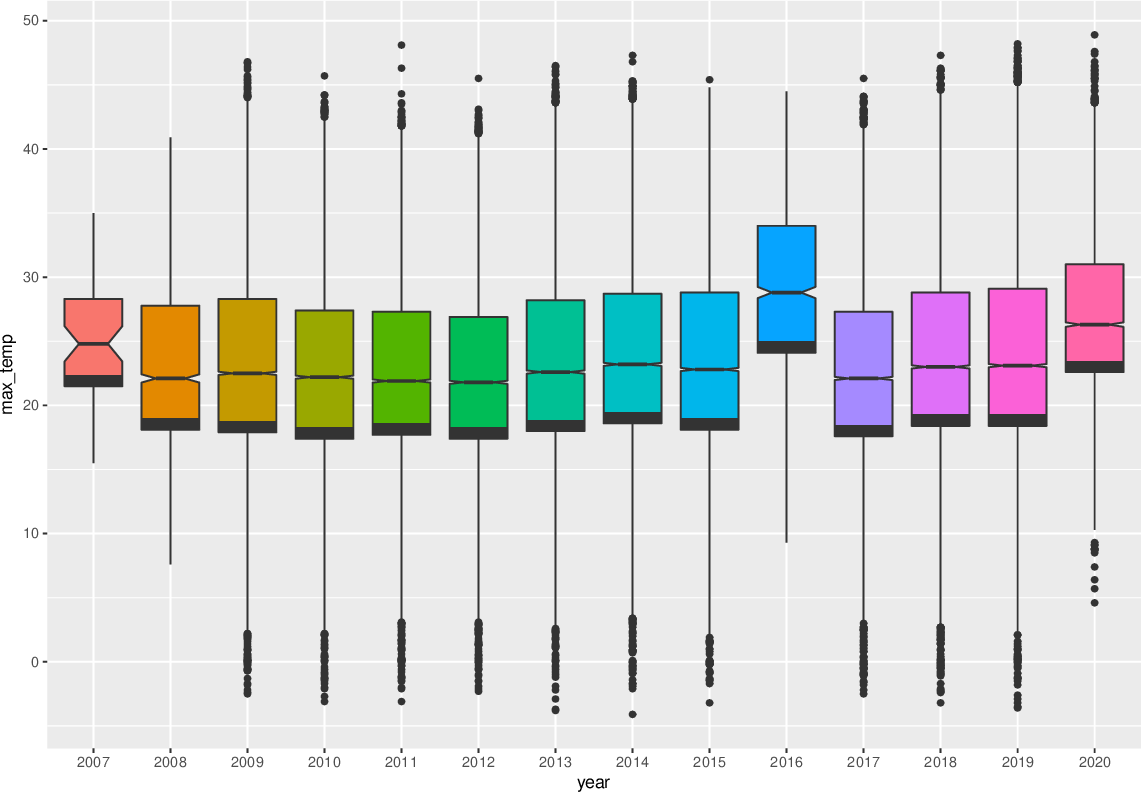Data Science Desktop Survival Guide by Graham WilliamsDesktop Survival Project Home Preface Data Science Introducing R R Constructs R Tasks R Strings R Read, Write, and Create Data Template Data Exploration Data Wrangling Data Visualisation Statistics ML Template ML Scenarios ML Activities ML Applications ML Algorithms Cluster Analysis Decision Trees Computer Vision Graph Data Privacy Literate Data Science Coding with Style Resources Bibliography Index

## Box Plot Distributions

Rawds                                                                    %>%   mutate(year=factor(format(ds\$date, "%Y")))                          %>%   ggplot(aes(x=year, y=max_temp, fill=year))                           +   geom_boxplot(notch=TRUE)                                             +   theme(legend.position="none") A box plot, also known as a box and whiskers plot, shows the median (the second quartile) within a box which extends to the first and third quartiles. We note that each quartile delimits one quarter of the dataset and hence the box itself contains half the dataset. Colour is added simply to improve the visual appeal of the plot rather than to convey new information. Since we include fill= we also turn off the otherwise included legend. Here we observe the overall change in the maximum temperature over the years. Notice the first and last plots which probably reflect truncated data, providing motivation to confirm this in the data, before making significant statements regarding these observations.